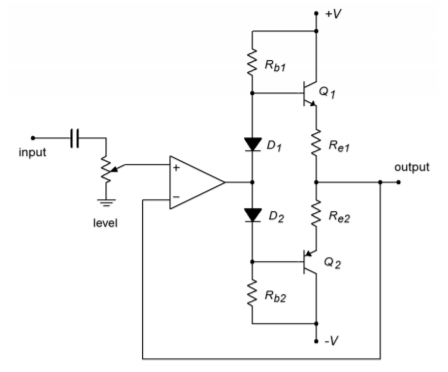# 7.10.4: Current Boosting

$$\newcommand{\vecs}{\overset { \rightharpoonup} {\mathbf{#1}} }$$ $$\newcommand{\vecd}{\overset{-\!-\!\rightharpoonup}{\vphantom{a}\smash {#1}}}$$$$\newcommand{\id}{\mathrm{id}}$$ $$\newcommand{\Span}{\mathrm{span}}$$ $$\newcommand{\kernel}{\mathrm{null}\,}$$ $$\newcommand{\range}{\mathrm{range}\,}$$ $$\newcommand{\RealPart}{\mathrm{Re}}$$ $$\newcommand{\ImaginaryPart}{\mathrm{Im}}$$ $$\newcommand{\Argument}{\mathrm{Arg}}$$ $$\newcommand{\norm}{\| #1 \|}$$ $$\newcommand{\inner}{\langle #1, #2 \rangle}$$ $$\newcommand{\Span}{\mathrm{span}}$$ $$\newcommand{\id}{\mathrm{id}}$$ $$\newcommand{\Span}{\mathrm{span}}$$ $$\newcommand{\kernel}{\mathrm{null}\,}$$ $$\newcommand{\range}{\mathrm{range}\,}$$ $$\newcommand{\RealPart}{\mathrm{Re}}$$ $$\newcommand{\ImaginaryPart}{\mathrm{Im}}$$ $$\newcommand{\Argument}{\mathrm{Arg}}$$ $$\newcommand{\norm}{\| #1 \|}$$ $$\newcommand{\inner}{\langle #1, #2 \rangle}$$ $$\newcommand{\Span}{\mathrm{span}}$$

As previously noted, general-purpose op amps produce a maximum output current of around 20 mA. This is sufficient for a wide variety of uses. If the load is less than about 1 k$$\Omega$$, the op amp will start to clip on the higher output signals. The average op amp cannot drive low impedance loads. A few examples of applications whose loads are inappropriate include distribution amplifiers, small audio power amplifiers such as a headphone amplifier, and small motors. This is most unfortunate, as we have already seen how useful these devices can be. There is a way out, though. It is possible to include a current gain stage right after the op amp. All that is needed is a simple class B or class AB push-pull follower. This follower will be able to produce the higher current required by low impedance loads. The op amp only needs to drive the follower stage. In order to increase system linearity and lower distortion, the follower can be placed inside of the op amp's feedback loop. Because the follower is noninverting, there is no problem with maintaining correct feedback (this assumes that the power devices used have a wider bandwidth than the op amp). An example of this is shown in Figure $$\PageIndex{1}$$.Figure $$\PageIndex{1}$$: Current boosting.

This circuit is typical of an electronic crossover or distribution amplifier (an electronic crossover design example is given in Chapter Eleven). This output circuit needs to drive relatively low impedances through long cable runs of perhaps several hundred feet. The excessive capacitance resulting from long cable runs increases the current demand above that of a purely resistive load.

Circuits like the one in Figure $$\PageIndex{1}$$ can produce currents of several hundred milliamps or more. Many times, small resistors are placed in the emitter or collector as a means of limiting maximum current or reducing distortion. The maximum output current limitation is a function of the class B devices. Some manufacturers offer current-boosting ICs to further simplify the design. The current-booster is a drop-in replacement for the class B follower. For very high current demands, Darlingtons or multi-stage designs may be required. It is even possible to provide voltage gain stages. Indeed, several consumer audio power amplifiers have been designed in exactly this way. In essence, the designers produce a discrete power amplifier and then “wrap it” within an op amp feedback loop.

## Computer Simulation

A basic current booster is simulated using Multisim in Figure $$\PageIndex{2}$$. In order to see secondary effects, the LF411 model has been chosen instead of the ideal device model.Figure $$\PageIndex{2a}$$: Current booster simulation schematic.

The circuit is configured for a voltage gain of unity, thus the 5 volt input signal should yield a 5 volt output. According to its data sheet, the short-circuit current of the LF411 is approximately 25 mA at room temperature. It is not capable of driving a 75 $$\Omega$$ load to 5 volts by itself. The Transient Analysis shows a full 5 volt output signal, indicating the effectiveness of the current boosting stage. Also, a close inspection of the output waveform shows no obvious forms of distortion such as the cross-over distortion typical of simple class B stages. This shows that keeping the class B stage within the feedback loop does indeed minimize distortion.Figure $$\PageIndex{2b}$$: Simulation of output waveform.

7.10.4: Current Boosting is shared under a CC BY-NC-SA license and was authored, remixed, and/or curated by James M. Fiore.